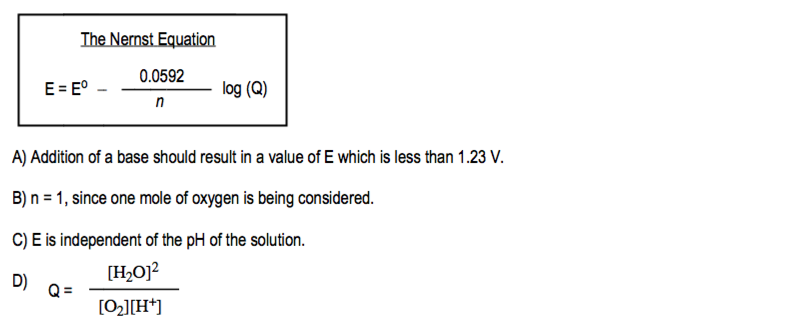# Problem: Consider this reaction.  4 e – + 4 H+ (aq) + O2 (g) ⇌ 2 H2O (l)     E° = 1.23 V Which statement is true if the hydrogen ion concentration is initially at 1.0 M and the initial pressure of oxygen gas is 1.0 atmosphere?

###### FREE Expert Solution
81% (187 ratings)
###### Problem Details

Consider this reaction.

4 e  + 4 H(aq) + O(g) ⇌ 2 H2O (l)     E° = 1.23 V

Which statement is true if the hydrogen ion concentration is initially at 1.0 M and the initial pressure of oxygen gas is 1.0 atmosphere?Frequently Asked Questions

What scientific concept do you need to know in order to solve this problem?

Our tutors have indicated that to solve this problem you will need to apply the The Nernst Equation concept. You can view video lessons to learn The Nernst Equation. Or if you need more The Nernst Equation practice, you can also practice The Nernst Equation practice problems.

What is the difficulty of this problem?

Our tutors rated the difficulty ofConsider this reaction.  4 e – + 4 H+ (aq) + O2 (g) ⇌ 2 H2O...as high difficulty.

How long does this problem take to solve?

Our expert Chemistry tutor, Jules took 4 minutes and 20 seconds to solve this problem. You can follow their steps in the video explanation above.

What professor is this problem relevant for?

Based on our data, we think this problem is relevant for Professor Bindell's class at UCF.